# Interaction Robot

ECE 5725 Fall 2022
Tianxin Yue(ty293), Xin Zhang(xz785), Jiahao Xu(jx98)

## Introduction

As advancements in robotics and AI continue to progress, the use of autonomous mobile robots has become increasingly common in the world. However, one of the biggest challenges facing these technologies is the ability of robots to effectively interact with humans. Our project aims to tackle this issue by developing a autonomous mobile robot that can be controlled through both gestures and voice commands.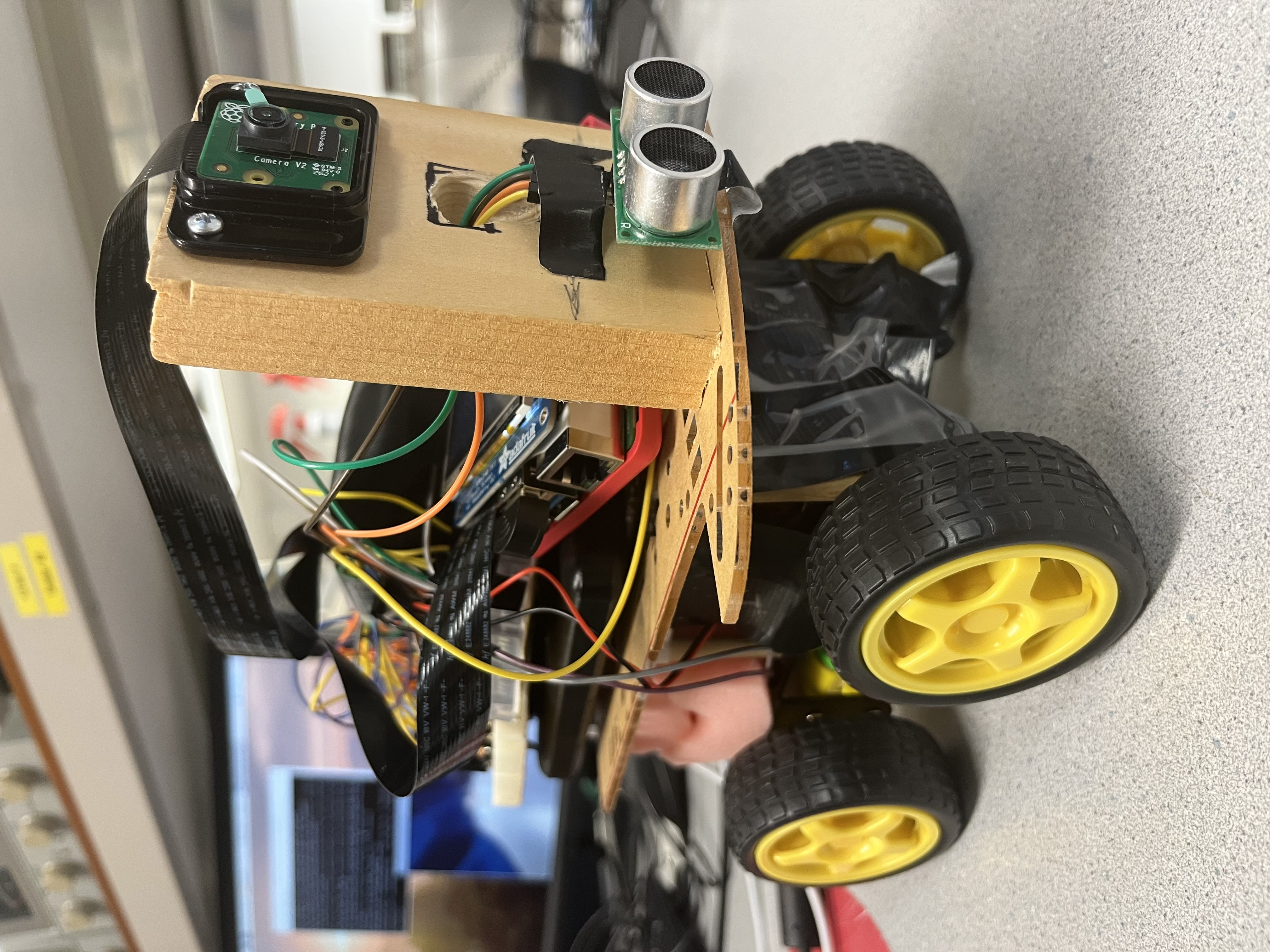## Project Objective

As advancements in robotics and AI continue to progress, the use of autonomous mobile robots has become increasingly common in the world. However, one of the biggest challenges facing these technologies is the ability of robots to effectively interact with humans. Our project aims to tackle this issue by developing a autonomous mobile robot that can be controlled through both gestures and voice commands.

• Designed the overall hardware layout for a motor-controlled, all-wheel drive autonomous mobile robot.
• Hand gesture interaction with four distinct gestures: distance keeping moving, stopping, turning left, and turning right implemented using Mediapipe and ropositional logic based encoding model.
• Voice recognition interaction with three commands: moving forward, turning left, and turning right implemented using the SpeechRecognition package in Python

## Design

Our project is composed of two main components: hardware and software. The hardware consists of a two-layer, all-wheel drive DC motor controlled chassis equipped with an RGB camera and an ultrasonic sensor for depth sensing. The software is further divided into three parts: gesture recognition, voice control, and software integration. In the gesture recognition module, we utilize the open-source Mediapipe package to identify hand gestures and extract key points from the hands. We have tested three different methods for translating these key points into target gestures: random forest, AutoML, and propositional-based encoding. We initially trained a random forest model using basic normalization techniques, but we found that it did not perform well due to a lack of data and overfitting. We then tried using AutoML, which is a state-of-the-art pipeline that automatically explores different ML models and performs auto-tuning. However, this method also proved ineffective in our case. As a result, we resorted to using heuristic methods that take advantage of the x-y coordinates of key points, and we found that this approach worked best for our purposes. In addition, we have integrated an ultrasonic sensor to obtain depth information about the hand gestures. This allows the robot to adjust its distance from the hand when moving forward or backward, maintaining a reasonable distance at all times. After developing the gesture recognition module, we turned our attention to the voice recognition part of the project. We used the SpeechRecognition package, which is a versatile library for performing speech recognition with multiple engines and APIs, both online and offline. This package allowed us to implement three commands: moving forward, turning left, and turning right. The final step is software integration, where we use the PiTFT buttons to switch between the gesture recognition and voice control modes, and to quit the script altogether. This allows for a seamless and user-friendly experience when interacting with the robot.

## Testing

### Robot car

In this project, we built a robot car based on Raspberry Pi4 and TB6612FNG dual-channel motor controller. We used Raspberry Pi GPIO as outputs, driving a DC motor using Pulse Width Modulation (PWM) from a GPIO output to control four motors. For this car, the left two motors share the same out pins, and the right two motors share the same out pins, that’s how we control four motors by using one motor controller. The graph below is the motor controller we used.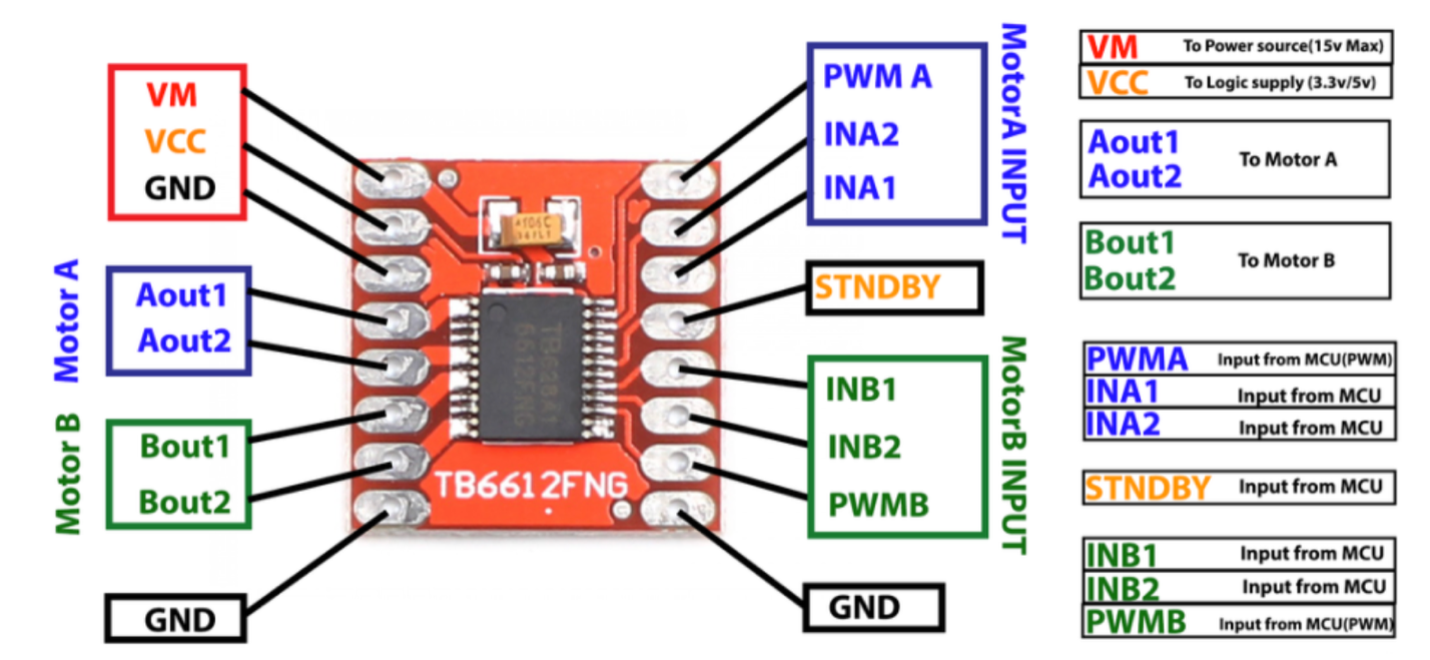After we connected the DC motor controller with Raspberry Pi and installed four DC motors, we tested the robot car by adjusting the duty cycle of PWM from GPIO pins to make the robot move forward, move backward, turn left, and turn right. The robot performed well on those four directions movement.

Then we installed the raspberry pi camera to the robot car, we used Raspberry Pi Camera Module V2 for this project. We tested the camera by using it to capture photos, record videos and display real-time window, and the camera works well on the robot car.

We also installed Ultrasonic Distance Sensor to measure the distance of an object with the robot car. The sensor works properly, and the distance between an object with the robot car can be displayed through the terminal window.The photo below is the final robot car we built.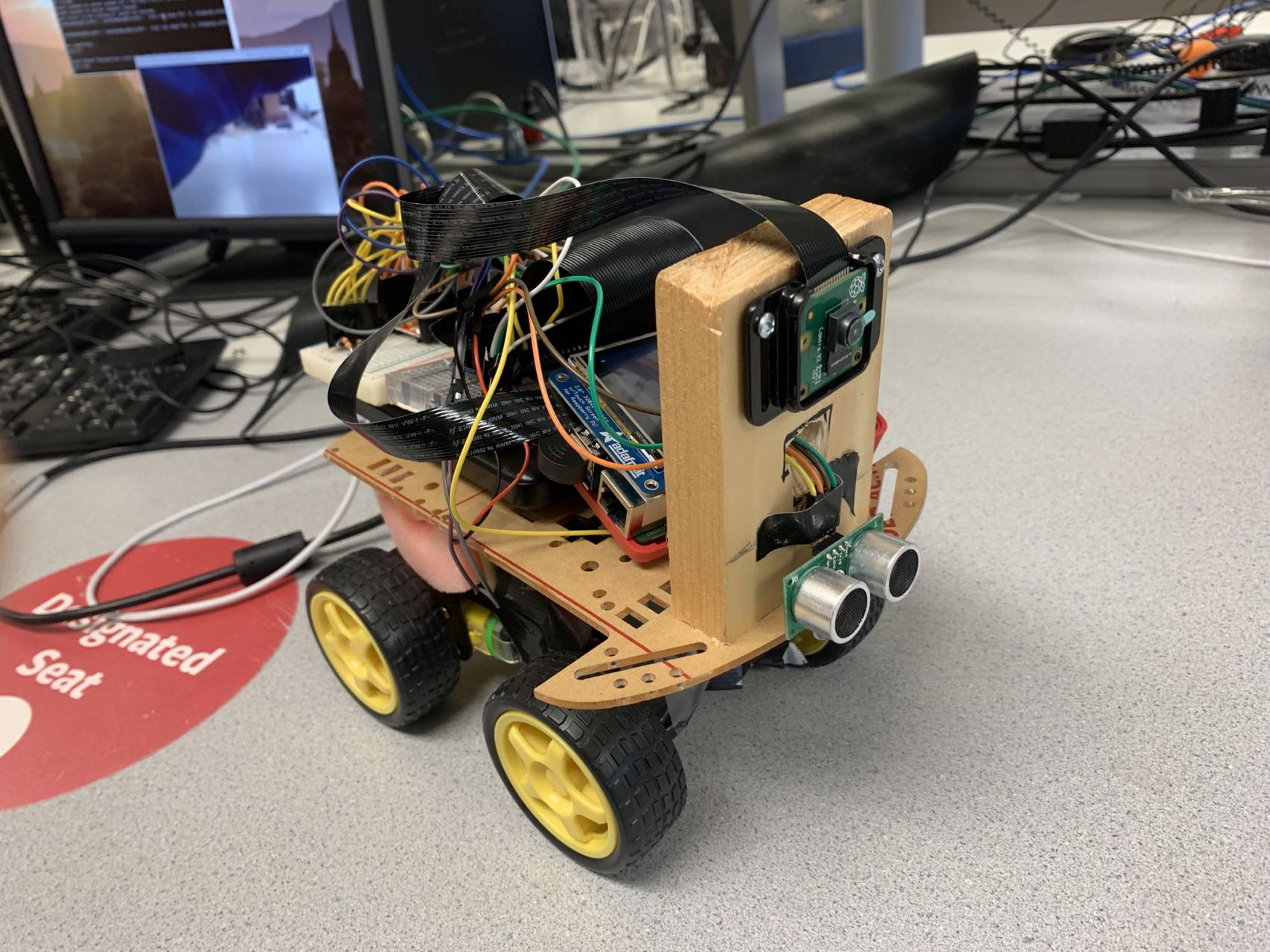### Hand gesture control

The robot car has two control mode, one is to use hand gesture to control the robot, and another is to use voice commands to control the robot. For the hand gesture control mode, we have tried three methods to make the robot recognize different kinds of hand gestures. The first method we used is to extract 21 key points of a hand using mediapipe and used the coordinates of those key points as the variables to train a random forest classifier machine learning model. The second method is that we used the same 21 key points to train an integrated machine learning model with different kinds of classifiers. It turns out that those two models both perform very well on the datasets we created but have an unstable performance on the real time video stream. To solve this problem, we used the third methods, that is to shrink the key points number, we only take points that are crucial to the four different kinds of hand gestures we tend to achieve. And we classified the hand gestures by identifying the relative coordinates of each point. The photo below is the testing result of the hand gestures control mode, the robot car successfully identifies four hand gestures.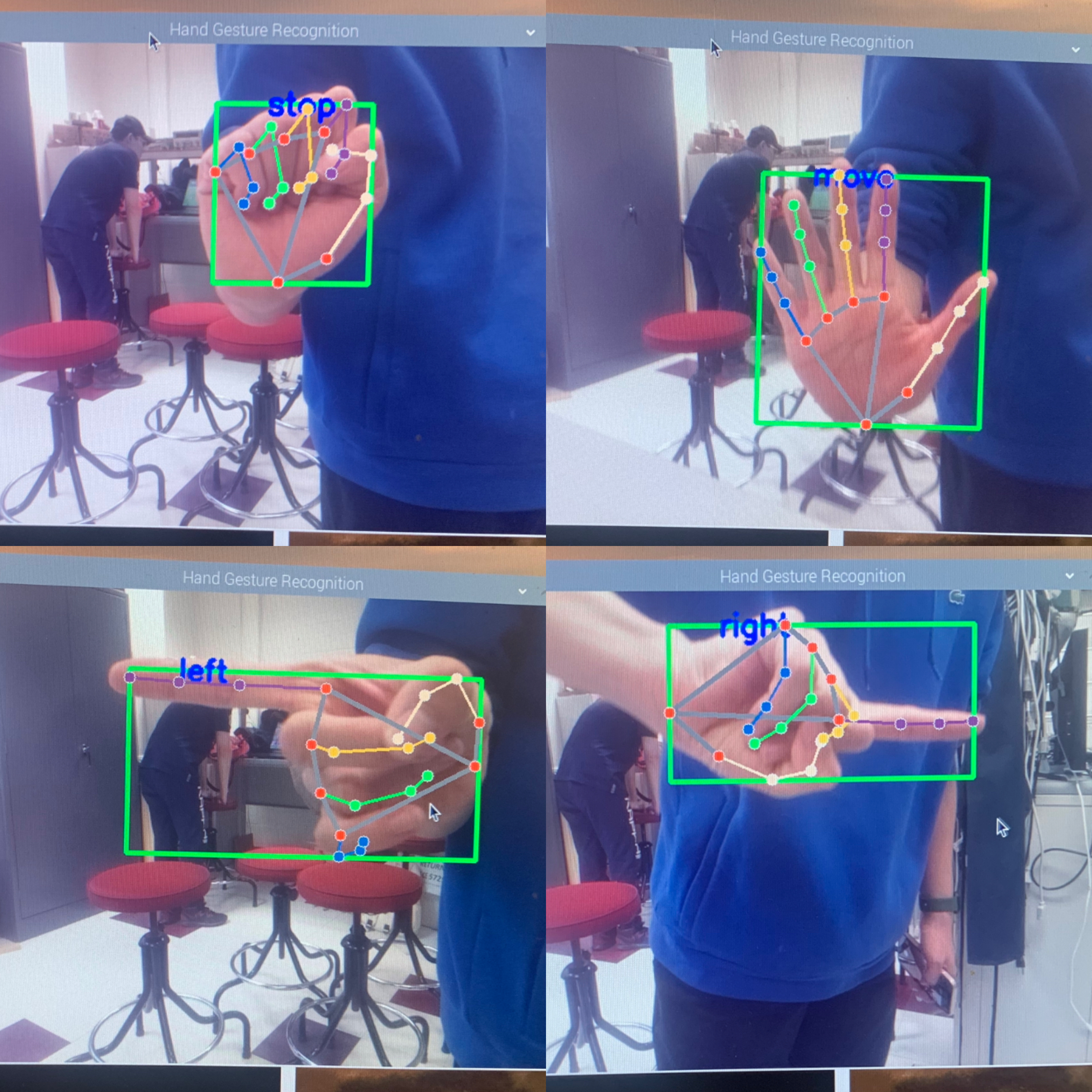### Voice command control

The second mode is to use the voice commands we said to control the robot car. To achieve this, we used a mini-USB microphone to record the sound we made and let the robot identify what commands we have made and moved according to those commands. We implemented this function by using speech recognition package in Python. We tested the voice command and the robot car three commands we said, including move forward, turn left and turn right.

## Result

Our goal is to build an interaction robot that users can control the movement of the robot by both hand gesture control as well as voice commands control. As far as the implementation, we installed the four-wheels robot car with camera and distance sensor, and we developed software in Python to control its movement by two modes in very high accuracy and efficient performance. The robot car can identify four kinds of hand gestures and three voice commands accurately and the robot is able to move according to those commands smoothly and quickly.

## Conclusion

As the implementation of our work, we built a robot that allow us to use our voice and hand gesture to control robots then we can easily operate them from a far distance which will reduce the use of long wires which can provide comfort to the users to interact with the robots easily and effectively. Also, the robot car controlled by voice and gesture makes it more useful in this present pandemic situation that we can reduce unnecessary human contact, this would be of great help to reduce human presence in the areas such as health care facilities and quarantine zones.

## Work Distribution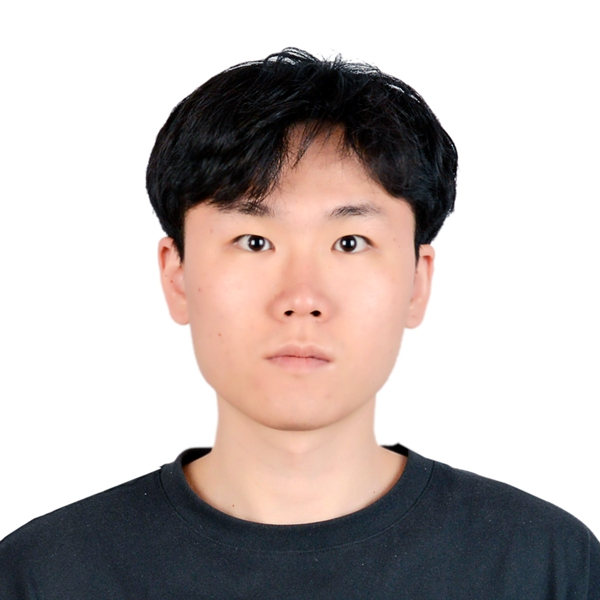### Xin Zhang

xz785@cornell.edu

Distance measure, Aultomatically boot, Construction of the model, Overall testing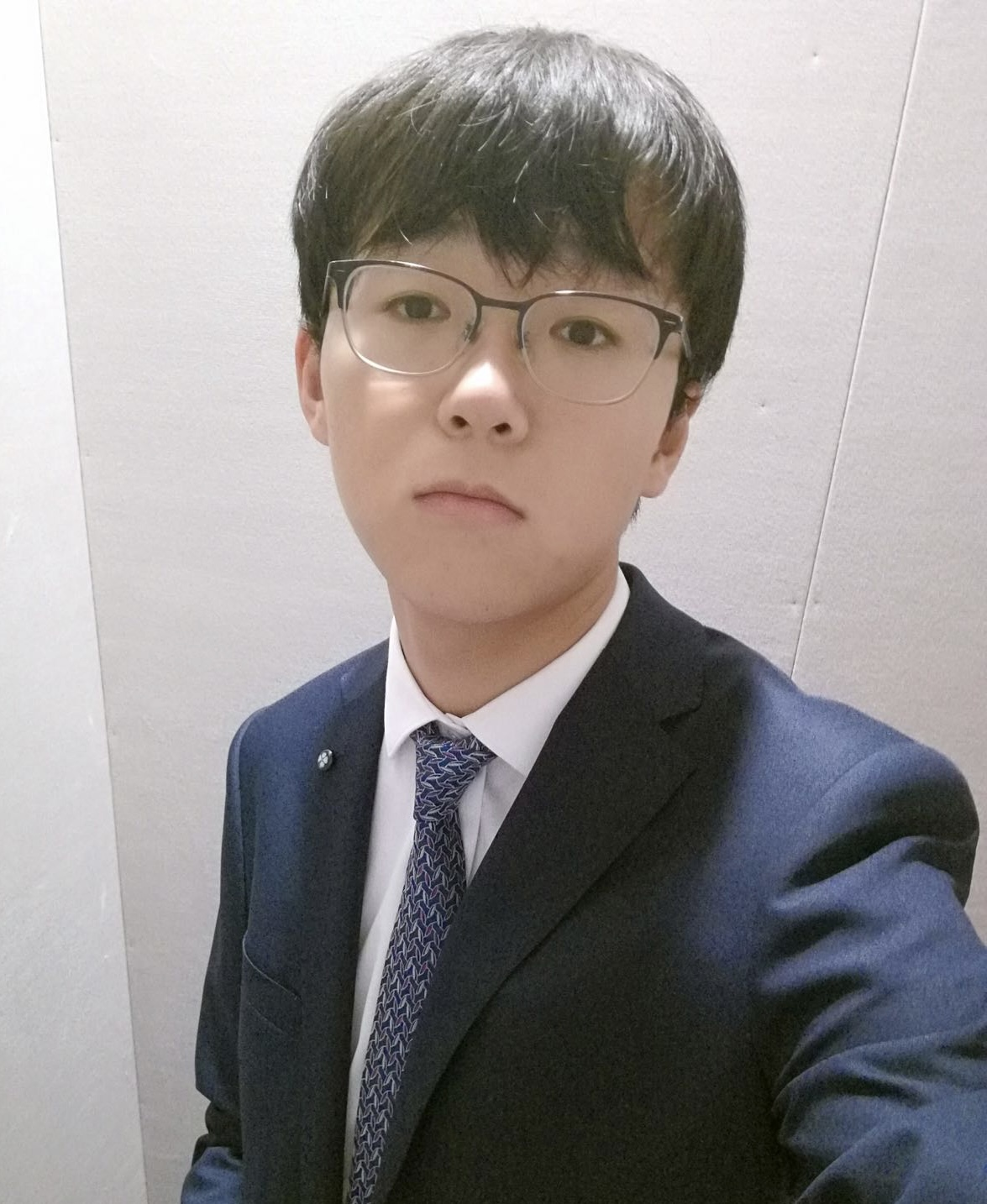### Jiahao Xu

jx98cornell.edu

Hand Gesture Recogntion, Overall testing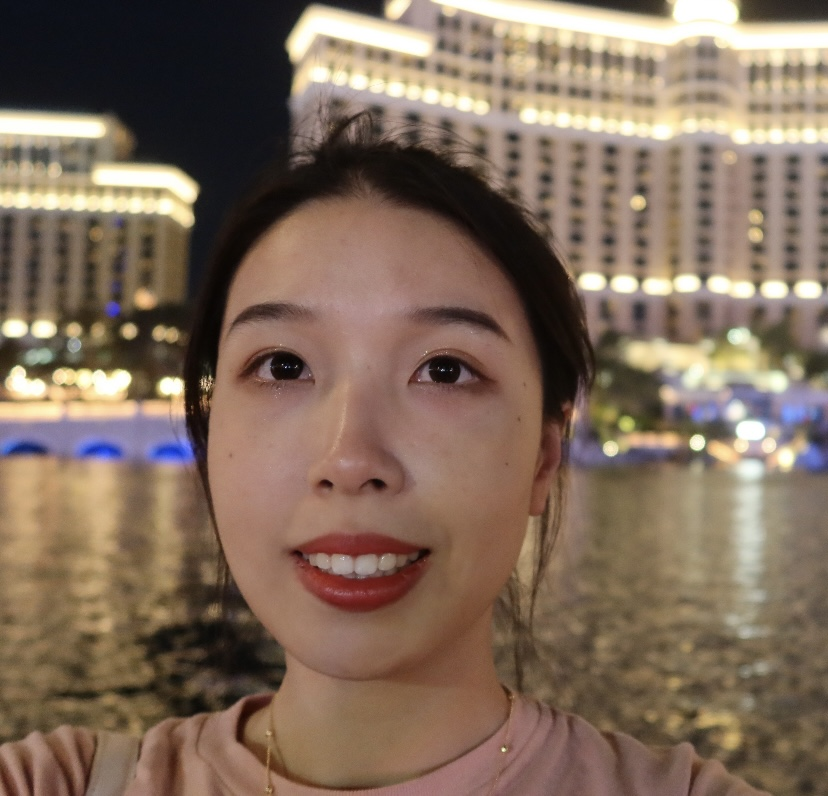### Tianxin Yue

ty293@cornell.edu

Hand gesture recognition model, speech recoginition model, robot car motor control

## Parts List

• Raspberry Pi \$35.00
• Raspberry Pi Camera V2 \$25.00
• Ultrasound Distance Sensor \$10.00
• Car Model \$18.00

## References

Mediepipe
Speech Recognition
Opencv

## Code Appendix

``````
import copy
import itertools
import pickle

import cv2 as cv
import numpy as np
import mediapipe as mp

import RPi.GPIO as GPIO
import speech_recognition as sr
import time
import collections

import pygame
import os
from pygame.locals import *
import time

def distance():
GPIO.output(GPIO_TRIGGER, True)

time.sleep(0.00001)
GPIO.output(GPIO_TRIGGER, False)

start_time = time.time()
stop_time = time.time()

while GPIO.input(GPIO_ECHO) == 0:
start_time = time.time()

while GPIO.input(GPIO_ECHO) == 1:
stop_time = time.time()

time_elapsed = stop_time - start_time

distance = (time_elapsed * 34300) / 2

return distance

def cal_bounding_rect(image, landmarks):
img_width = image.shape
img_height = image.shape

landmark_array = np.empty((0, 2), int)

for _, landmark in enumerate(landmarks.landmark):
landmark_x = min(int(landmark.x * img_width), img_width - 1)
landmark_y = min(int(landmark.y * img_height), img_height - 1)

landmark_point = [np.array((landmark_x, landmark_y))]
landmark_array = np.append(landmark_array, landmark_point, axis=0)

x, y, w, h = cv.boundingRect(landmark_array)

return [x, y, x + w, y + h]

def cal_landmark_list(image, landmarks):
img_width = image.shape
img_height = image.shape
landmark_point = []
for _, landmark in enumerate(landmarks.landmark):
landmark_x = min(int(landmark.x * img_width), img_width - 1)
landmark_y = min(int(landmark.y * img_height), img_height - 1)
landmark_point.append([landmark_x, landmark_y])

return landmark_point

def preprocess_landmark(landmark_list):
temp_lm_list = copy.deepcopy(landmark_list)

base_x = 0
base_y = 0

for index, landmark_point in enumerate(temp_lm_list):
if index == 0:
base_x, base_y = landmark_point, landmark_point

temp_lm_list[index] = temp_lm_list[index] - base_x
temp_lm_list[index] = temp_lm_list[index] - base_y

temp_lm_list = list(itertools.chain.from_iterable(temp_lm_list))

max_value = max(list(map(abs, temp_lm_list)))

def normalize_(n):
return n / max_value

temp_lm_list = list(map(normalize_, temp_lm_list))

return temp_lm_list

def clockwise(p, dc, in1, in2):
p.ChangeDutyCycle(dc)
GPIO.output(in1, GPIO.HIGH)
GPIO.output(in2, GPIO.LOW)
return None

def counterclockwise(p, dc, in1, in2):
p.ChangeDutyCycle(dc)
GPIO.output(in2, GPIO.HIGH)
GPIO.output(in1, GPIO.LOW)
return None

def stop(p):
p.ChangeDutyCycle(0)
return None

# --------------------Initialize--------------
GPIO.setmode(GPIO.BCM)

GPIO_TRIGGER = 26
GPIO_ECHO = 19

GPIO.setup(GPIO_TRIGGER, GPIO.OUT)
GPIO.setup(GPIO_ECHO, GPIO.IN)

#os.putenv('SDL_VIDEODRIVER', 'fbcon')
#os.putenv('SDL_FBDEV', '/dev/fb1')
#os.putenv('SDL_MOUSEDRV', 'TSLIB')
#os.putenv('SDL_MOUSEDEV', '/dev/input/touchscreen')

GPIO.setup(27, GPIO.IN, pull_up_down=GPIO.PUD_UP)
GPIO.setup(22, GPIO.IN, pull_up_down=GPIO.PUD_UP)
GPIO.setup(23, GPIO.IN, pull_up_down=GPIO.PUD_UP)

'''
start_time = time.time()
pygame.init()
# Control Mode
hand_control = False
voice_control = False
# pygame.mouse.set_visible(True)
WHITE = 255, 255, 255
BLACK = 0, 0, 0
screen = pygame.display.set_mode((320, 240))
my_font = pygame.font.Font(None, 50)
my_buttons = {'Hand Control': (150, 200), 'Voice Control': (150, 100)}
screen.fill(BLACK)
my_button_rect = {}
for my_text, text_pos in my_buttons.items():
text_surface = my_font.render(my_text, True, WHITE)
rect = text_surface.get_rect(center=text_pos)
screen.blit(text_surface, rect)
my_button_rect[my_text] = rect
pygame.display.flip()
'''

#stk_voice = collections.deque(['move', 'left', 'right'])
stk_voice = collections.deque()
stk = collections.deque(['stop', 'stop', 'stop', 'stop'])
# GPIO.setmode(GPIO.BCM)
GPIO.setwarnings(False)
AIN1 = 5
AIN2 = 6
PWMA = 13
BIN1 = 20
BIN2 = 21
PWMB = 12
GPIO.setmode(GPIO.BCM)
GPIO.setup(PWMA, GPIO.OUT)
GPIO.setup(AIN1, GPIO.OUT)
GPIO.setup(AIN2, GPIO.OUT)
GPIO.setup(PWMB, GPIO.OUT)
GPIO.setup(BIN1, GPIO.OUT)
GPIO.setup(BIN2, GPIO.OUT)
frequency = 50
stop_dc = 0
half_dc = 20
full_dc = 40
pA = GPIO.PWM(PWMA, frequency)
pA.start(0)
pB = GPIO.PWM(PWMB, frequency)
pB.start(0)

# -----------------Button Control--------------
code_run = True
hand_control = False
voice_control = False
while code_run:
if not GPIO.input(27):
code_run = False

if not GPIO.input(22):
print("Hand Control Mode")
hand_control = True
voice_control = False

if not GPIO.input(23):
print("Voice Control Mode")
hand_control = False
voice_control = True

if hand_control:
cap = cv.VideoCapture(0)
mp_drawing = mp.solutions.drawing_utils
mp_drawing_styles = mp.solutions.drawing_styles

filename = 'rand_forest.sav'

mp_hands = mp.solutions.hands
hands = mp_hands.Hands(
max_num_hands=1,
min_detection_confidence=0.7,
min_tracking_confidence=0.5
)

previous_label = 'stop'

while hand_control:
gesture_label = 'none'
if not GPIO.input(27):
code_run = False
hand_control = False
voice_control = False
cap.release()
if not GPIO.input(23):
print("Voice Control Mode")
hand_control = False
voice_control = True
cap.release()

key = cv.waitKey(1)
if key == 27:
break

# camera capture

if not ret:
continue

image = cv.flip(image, -1)
debug_image = copy.deepcopy(image)

image = cv.cvtColor(image, cv.COLOR_BGR2RGB)
image.flags.writeable = False
results = hands.process(image)
image.flags.writeable = True

if results.multi_hand_landmarks:
for hand_landmarks in results.multi_hand_landmarks:

brect = cal_bounding_rect(debug_image, hand_landmarks)
landmark_list = cal_landmark_list(debug_image, hand_landmarks)
array = []
array_x = []
array_y = []
for i, lm in enumerate(hand_landmarks.landmark):
yPos = int(lm.y * image.shape)
xPos = int(lm.x * image.shape)
array_x.append(xPos)
array_y.append(yPos)
array.append(xPos)
array.append(yPos)

if len(array_y) == 0:
continue
# print(array_x)
# print(array_y)
if min(array_x, array_x, array_x) == min(array_x) and max(array_x, array_x,
array_x) == max(array_x):
print("LEFT")
gesture_label = "left"
elif array_y == max(array_y) and array_y <= array_y and array_y <= array_y and array_y[
16] <= array_y:
print("Move")
gesture_label = "move"
elif array_y == max(array_y) and array_y >= array_y and array_y >= array_y and array_y[
16] >= array_y:
print("Stop")
gesture_label = "stop"
elif array_y != max(array_y) and array_x > array_x:
print("RIGHT")
gesture_label = "right"

debug_image = cv.rectangle(debug_image, (brect, brect), (brect, brect),
(0, 255, 0), 4)

text_position = (brect + 50, brect + 10)
debug_image = cv.putText(debug_image, gesture_label, text_position, cv.FONT_HERSHEY_SIMPLEX, 1,
(255, 0, 0), 3)

mp_drawing.draw_landmarks(
debug_image,
hand_landmarks,
mp_hands.HAND_CONNECTIONS,
mp_drawing_styles.get_default_hand_landmarks_style(),
mp_drawing_styles.get_default_hand_connections_style())

# print(gesture_label)

cv.imshow('Hand Gesture Recognition', debug_image)
stk.popleft()
stk.append(gesture_label)
if stk == stk and stk == stk and stk == stk:
if stk == 'stop':
stop(pA)
stop(pB)
print('stop')

elif (stk == 'move'):
dist = distance()
print("Measured Distance = {:.2f} cm".format(dist))
if(dist<30):
counterclockwise(pA, full_dc, AIN1, AIN2)
counterclockwise(pB, full_dc, BIN1, BIN2)
print('distance less than 30, back')
else:
clockwise(pA, full_dc, AIN1, AIN2)
clockwise(pB, full_dc, BIN1, BIN2)
print('distance more than 30, move')

elif (stk == 'left'):
clockwise(pA, 2*full_dc, AIN1, AIN2)
counterclockwise(pB, 2*full_dc, BIN1, BIN2)
print('left')
time.sleep(0.2)
clockwise(pA, stop_dc, AIN1, AIN2)
clockwise(pB, stop_dc, BIN1, BIN2)
elif (stk == 'right'):
counterclockwise(pA, 2*full_dc, AIN1, AIN2)
clockwise(pB, 2*full_dc, BIN1, BIN2)
print('right')
time.sleep(0.2)
clockwise(pA, stop_dc, AIN1, AIN2)
clockwise(pB, stop_dc, BIN1, BIN2)

while voice_control:
clockwise(pA, stop_dc, AIN1, AIN2)
clockwise(pB, stop_dc, BIN1, BIN2)
if not GPIO.input(27):
code_run = False
hand_control = False
voice_control = False

if not GPIO.input(22):
print("Hand Control Mode")
hand_control = True
voice_control = False

#key = cv.waitKey(1)
#if key == 27:
#break

# camera capture

r = sr.Recognizer()
speech = sr.Microphone(device_index=2)
with speech as source:
print("say something!…")
#print("step1")
#print("step2")
#            if not GPIO.input(27):
#                print("button 27")
#                code_run = False
#                hand_control = False
#                voice_control = False
#                print(code_run,hand_control,voice_control)
#
#                break
audio = r.listen(source)
#print("step3")
try:
#print("try")
stk_voice.append(recog)
print("You said: " + recog)
except sr.UnknownValueError:
print("Google Speech Recognition could not understand audio")
except sr.RequestError as e:
print("Could not request results from Google Speech Recognition service; {0}".format(e))
except speech_recognition.WaitTimeoutError as e:
print("other reason")
except Exception as e:
print("error")
string_left = ["left","lef","let","up","lock"]
string_right = ["right","rice","but"]

if len(stk_voice) >= 1:
for each in string_move:
if each in recog.lower():

clockwise(pA, full_dc, AIN1, AIN2)
clockwise(pB, full_dc, BIN1, BIN2)
time.sleep(1)
clockwise(pA, stop_dc, AIN1, AIN2)
clockwise(pB, stop_dc, BIN1, BIN2)
break

for each in string_left:
if each in recog.lower():
clockwise(pA, 2 * full_dc, AIN1, AIN2)
counterclockwise(pB, 2 * full_dc, BIN1, BIN2)
print('left')
time.sleep(1)
clockwise(pA, stop_dc, AIN1, AIN2)
clockwise(pB, stop_dc, BIN1, BIN2)
break
for each in string_right:
if each in recog.lower():
counterclockwise(pA, 2 * full_dc, AIN1, AIN2)
clockwise(pB, 2 * full_dc, BIN1, BIN2)
print('right')
time.sleep(1)
clockwise(pA, stop_dc, AIN1, AIN2)
clockwise(pB, stop_dc, BIN1, BIN2)

stk_voice.popleft()

print("stop time")
for i in range(10):
if not GPIO.input(27):
print("button 27")
code_run = False
hand_control = False
voice_control = False
if not GPIO.input(22):
print("Hand Control Mode")
hand_control = True
voice_control = False
time.sleep(0.2)

#cap.release()

pA.stop()
pB.stop()

GPIO.output(AIN2, GPIO.LOW)
GPIO.output(AIN1, GPIO.LOW)
GPIO.output(BIN2, GPIO.LOW)
GPIO.output(BIN1, GPIO.LOW)

GPIO.cleanup()
``````